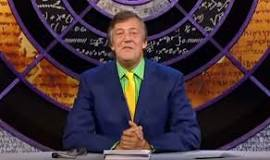# Maths trickHere’s a Maths trick a friend of mine saw on QI. Who knows? It might make addition and subtraction just a little bit more fun!

1. Find a book and write down the ninth word on p108, eg ‘becoming’.
2. Ask someone to write down a number with three different digits in it, eg 321.
3. Ask him to reverse it and take the smaller number away from the larger, eg 321 – 123 = 198.
4. Ask him to write down the answer and, again, reverse it, eg 891.
5. Ask him to add the two new numbers together, eg 198 + 891 = 1089.
6. Ask him to find the ninth word on p108 of the book, eg ‘becoming’.
7. Reveal the same word you wrote down earlier!

The trick is that the answer is always going to be 1089, whatever the number you first think of, so it should work every time – unless there’s a problem with the Maths!